# Chapter 19: Transparency

## 19.1 PdfGState Object: Overview

19.1.1 PdfGState As a Graphics State Object

A graphics state is a central notion in the PDF format. The current graphics state is made up of many parameters affecting painting operations such as the current stroke and fill colors, line width, font, and many others. With AspPDF, the graphics state is modified via various properties and methods of the PdfCanvas object such as SetFillColor or LineWidth. See Section 4.4 - Managing Graphics State for a detailed discussion of the graphics state.

As an alternative to setting individual parameters via separate operators, PDF also allows multiple parameters of the current graphics state to be modified in a single operation, via the graphics state object referred to in the PDF specifications as ExtGState. An instance of the graphics state object is created and populated with multiple parameters and then selected in a single step, and can be reused if necessary. While using the graphics state object seems redundant, there are in fact certain graphics state parameters that can only be specified via a graphics state object and not any other way. Transparency-related parameters are among those that require a graphics state object. As of Version 3.1, in order for AspPDF to support transparency, its object model has been expanded to include a new object, PdfGState, which represents both a PDF ExtGState object, and a transparency group described in the following subsection.

An instance of the PdfGState object is created via the PdfDocument method CreateGState. This method expects a single argument, a PdfParam object or parameter string specifying various graphics state parameters including those related to transparency. To activate this PdfGState object, it is passed to the PdfCanvas method SetGState.

The following code snippet draws a semi-transparent text over a PDF page. The blend mode (BlendMode parameter) is set to Normal (1). Blend modes are covered in detail below. Both the stroke and fill transparency values (Alpha and FillAlpha parameters) are set to 0.2 (20%.)

Set PDF = Server.CreateObject("Persits.PDF")
Set Doc = PDF.OpenDocument("c:\path\doc.pdf")
Set GState = Doc.CreateGState("BlendMode=1; Alpha=0.2; FillAlpha=0.2")
Set Page = Doc.Pages(1)
Page.Canvas.SetGState GState
Page.Canvas.DrawText "DRAFT", "x=-40; y=170; angle=52; size=240; color=blue", Doc.Fonts("Helvetica")
Doc.Save "c:\path\out.pdf", False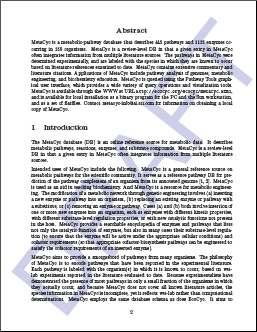19.1.2 PdfGState As a Transparency Group Object

In addition to representing a graphics state object, the PdfGState object has also been assigned the task of representing a transparency group, an important entity in the PDF transparency model. Transparency groups are described in detail below.

To create a transparency group rather than a graphics state object, the CreateGState method must be called with the Group=True parameter. Other group-related parameters can be specified as well. When the Group parameter is set to True, all graphics state-related parameters are ignored. The instance of the PdfGState object representing a transparency group can then be assigned to a PdfGraphics or PdfPage object via the method SetGroup, for example:

...
Set Group = Doc.CreateGState("Group=True")
Set Graphics = Doc.CreateGraphics("left=0; bottom=0; right=200; top=200")
Graphics.SetGroup Group
...

The meaning of this code snippet will be explained below.

## 19.2 Blend Mode

From the Adobe PDF Reference: "The original Adobe imaging model paints objects (fills, strokes, text, and images) opaquely onto a page. The color of the page at any point is that of the topmost enclosing object, disregarding any previous objects it may overlap... Under the transparency imaging model, all of the objects on a page can potentially contribute to the result. Objects at a given point can be thought of as forming a transparency stack (or stack for short). The objects are arranged from bottom to top in the order in which they are specified. The color of the page at each point is determined by combining the colors of all enclosing objects in the stack according to compositing rules defined by the transparency model... A given object is composited with a backdrop. Ordinarily, the backdrop consists of the stack of all objects that have been specified previously. The result of compositing is then treated as the backdrop of the next object."

NOTE: A comprehensive description of the PDF Transparency Model is beyond the scope of this user manual. Please refer to the Adobe PDF Reference for detailed information on this complex subject.

Under the PDF transparency model, when an object is painted, its color and alpha values are combined with the current backdrop's color and alpha values to form a new current color and alpha values. The new values are computed according to the basic color compositing formula which takes into account the source color (Cs), backdrop color (Cb), source alpha (αs), backdrop alpha (αb), and a blend function B(Cb, Cs) which specifies how the source and backdrop colors are combined in the painting operation: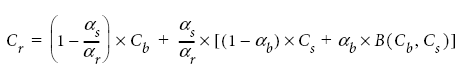The blend function determines the blend mode of the painting operation and there are 16 pre-defined blend modes to choose from. For example, under the Normal blend mode the blend function is simply

B(Cb, Cs) = Cs

and under the Multiply mode it is

B(Cb, Cs) = Cb x Cs

The blend mode is specified via the BlendMode parameter of the PdfDocument.CreateGState method. The valid values are 1 to 16.

The following table summarizes all the available blend modes and their effects when the picture of a duck is painted on top of the picture of a rainbow.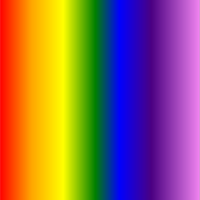Name
BlendMode
Effect
Name
BlendMode
Effect
Normal
1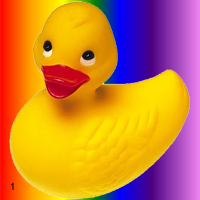Multiply
2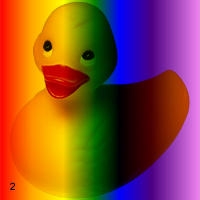Screen
3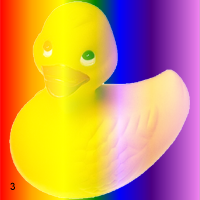Overlay
4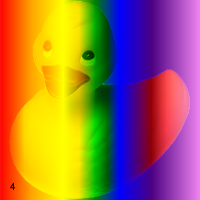Darken
5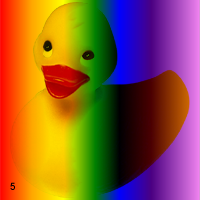Lighten
6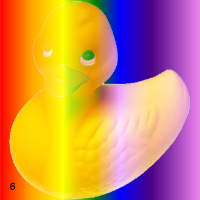ColorDodge
7ColorBurn8
8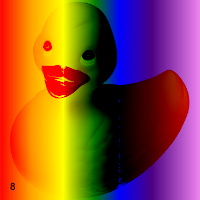HardLight
9SoftLight
10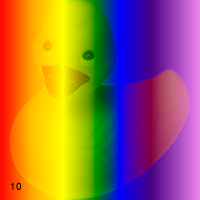Difference11
11Exclusion
12Hue
13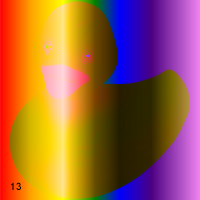Saturation
14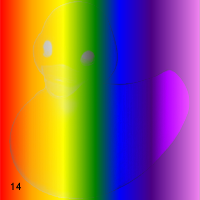Color
15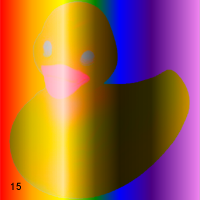Luminosity
16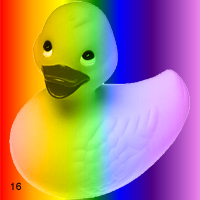## 19.3 Transparency Groups

19.3.1 Groups: Overview

One or more consecutive objects in a stack can be collected together into a transparency group, or simply group. The group as a whole can have various properties that modify the compositing behavior of objects within the group and their interactions with its backdrop. An additional blend mode and alpha can also be associated with the group as a whole and used when compositing it with its backdrop. Groups can be nested within other groups.

With AspPDF, a transparency group is implemented by a PdfGraphics object linked to a PdfGState object representing a group, as follows:

Set Group = Doc.CreateGState("Group=true; <other parameters>")
Set Graph = Doc.CreateGraphics("<list of parameters>")
Graph.SetGroup Group

The following four images demonstrate the effects of transparency grouping. In the upper two figures, three colored circles are painted as independent objects with no grouping. At the upper left, the three objects are painted opaquely; each completely replaces its backdrop (including previously painted objects) with its own color. At the upper right, the same three independent objects are painted with an opacity of 0.5, causing them to composite with each other and with the gray and white background. In the lower two figures, the three objects are combined as a transparency group. At the lower-left, the individual objects have an opacity of 1.0 within the group, but the group as a whole is painted in the Normal blend mode (BlendMode=1) with an opacity of 0.5 (FillAlpha=0.5). At the lower right, the objects have an opacity of 0.5 within the group and thus compose with each other. The group as a whole is painted against the backdrop with an opacity of 1.0 but in a different blend mode, HardLight (BlendMode=9), producing a different visual effect.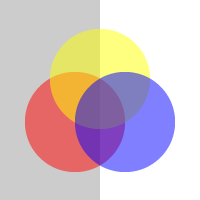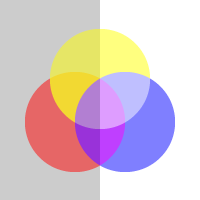Below is the corresponding AspPDF script:

' Common part: draw background
Set PDF = Server.CreateObject("Persits.PDF")
Set Doc = PDF.CreateDocument
Page.Canvas.SetFillColor 0.8, 0.8, 0.8
Page.Canvas.FillRect 0, 0, page.width / 2, page.height
Page.Canvas.SetFillColor 1, 1, 1
Page.Canvas.FillRect page.width / 2, 0, page.width, page.height

Page.Canvas.SaveState
Page.Canvas.SetCTM 1, 0, 0, 1, 206, 600
Call UpperLeft
Page.Canvas.RestoreState

Page.Canvas.SaveState
Page.Canvas.SetCTM 1, 0, 0, 1, 206, 400
Call UpperRight
Page.Canvas.RestoreState

Page.Canvas.SaveState
Page.Canvas.SetCTM 1, 0, 0, 1, 206, 200
Call LowerLeft
Page.Canvas.RestoreState

Page.Canvas.SaveState
Page.Canvas.SetCTM 1, 0, 0, 1, 206, 0
Call LowerRight
Page.Canvas.RestoreState

' Save document, the Save method returns generated file name
Filename = Doc.Save( Server.MapPath("transp.pdf"), False )

' Upper-left: no grouping or transparency
Sub UpperLeft
Set Circ1 = Doc.CreateGraphics("left=0; bottom=0; right=100; top=100")
Circ1.Canvas.SetFillColor 1, 0, 0
Circ1.Canvas.FillEllipse 50, 50, 50, 50

Set Circ2 = Doc.CreateGraphics("left=0; bottom=0; right=100; top=100")
Circ2.Canvas.SetFillColor 1, 1, 0
Circ2.Canvas.FillEllipse 50, 50, 50, 50

Set Circ3 = Doc.CreateGraphics("left=0; bottom=0; right=100; top=100")
Circ3.Canvas.SetFillColor 0, 0, 1
Circ3.Canvas.FillEllipse 50, 50, 50, 50

Set Graph = Doc.CreateGraphics("left=0; bottom=0; right=200; top=200")
Graph.Canvas.DrawGraphics Circ1, "x=25; y=28"
Graph.Canvas.DrawGraphics Circ2, "x=50; y=71"
Graph.Canvas.DrawGraphics Circ3, "x=75; y=28"

Page.Canvas.DrawGraphics Graph, "x=0; y=0"
End Sub

' Upper-right: no grouping, 0.5 opacity
Sub UpperRight
Set Circ1 = Doc.CreateGraphics("left=0; bottom=0; right=100; top=100")
Circ1.Canvas.SetFillColor 1, 0, 0
Circ1.Canvas.FillEllipse 50, 50, 50, 50

Set Circ2 = Doc.CreateGraphics("left=0; bottom=0; right=100; top=100")
Circ2.Canvas.SetFillColor 1, 1, 0
Circ2.Canvas.FillEllipse 50, 50, 50, 50

Set Circ3 = Doc.CreateGraphics("left=0; bottom=0; right=100; top=100")
Circ3.Canvas.SetFillColor 0, 0, 1
Circ3.Canvas.FillEllipse 50, 50, 50, 50

Set Graph = Doc.CreateGraphics("left=0; bottom=0; right=200; top=200")
Set GState = Doc.CreateGState("BlendMode=1; FillAlpha=0.5")
Graph.Canvas.SetGState GState
Graph.Canvas.DrawGraphics Circ1, "x=25; y=28"
Graph.Canvas.DrawGraphics Circ2, "x=50; y=71"
Graph.Canvas.DrawGraphics Circ3, "x=75; y=28"

Page.Canvas.DrawGraphics Graph, "x=0; y=0"
End Sub

' Lower-left: grouping, no opacity within group, 0.5 opacity for entire group
Sub LowerLeft
Set Circ1 = Doc.CreateGraphics("left=0; bottom=0; right=100; top=100")
Circ1.Canvas.SetFillColor 1, 0, 0
Circ1.Canvas.FillEllipse 50, 50, 50, 50

Set Circ2 = Doc.CreateGraphics("left=0; bottom=0; right=100; top=100")
Circ2.Canvas.SetFillColor 1, 1, 0
Circ2.Canvas.FillEllipse 50, 50, 50, 50

Set Circ3 = Doc.CreateGraphics("left=0; bottom=0; right=100; top=100")
Circ3.Canvas.SetFillColor 0, 0, 1
Circ3.Canvas.FillEllipse 50, 50, 50, 50

Set Group = Doc.CreateGState( "Group=true")
Set Graph = Doc.CreateGraphics("left=0; bottom=0; right=200; top=200")
Graph.SetGroup Group
Graph.Canvas.DrawGraphics Circ1, "x=25; y=28"
Graph.Canvas.DrawGraphics Circ2, "x=50; y=71"
Graph.Canvas.DrawGraphics Circ3, "x=75; y=28"

Set GState = Doc.CreateGState("BlendMode=1; FillAlpha=0.5")
Page.Canvas.SetGState GState
Page.Canvas.DrawGraphics Graph, "x=0; y=0"
End Sub

' Lower-right: grouping, 0.5 opacity within group, no opacity for entire group, HardLight
Sub LowerRight
Set GState = Doc.CreateGState("BlendMode=1; FillAlpha=0.5")

Set Circ1 = Doc.CreateGraphics("left=0; bottom=0; right=100; top=100")
Circ1.Canvas.SetGState GState
Circ1.Canvas.SetFillColor 1, 0, 0
Circ1.Canvas.FillEllipse 50, 50, 50, 50

Set Circ2 = Doc.CreateGraphics("left=0; bottom=0; right=100; top=100")
Circ2.Canvas.SetGState GState
Circ2.Canvas.SetFillColor 1, 1, 0
Circ2.Canvas.FillEllipse 50, 50, 50, 50

Set Circ3 = Doc.CreateGraphics("left=0; bottom=0; right=100; top=100")
Circ3.Canvas.SetGState GState
Circ3.Canvas.SetFillColor 0, 0, 1
Circ3.Canvas.FillEllipse 50, 50, 50, 50

Set Group = Doc.CreateGState( "Group=true")
Set Graph = Doc.CreateGraphics("left=0; bottom=0; right=200; top=200")
Graph.SetGroup Group
Graph.Canvas.DrawGraphics Circ1, "x=25; y=28"
Graph.Canvas.DrawGraphics Circ2, "x=50; y=71"
Graph.Canvas.DrawGraphics Circ3, "x=75; y=28"

Set GState2 = Doc.CreateGState("BlendMode=9; FillAlpha=1")
Page.Canvas.SetGState GState2
Page.Canvas.DrawGraphics Graph, "x=0; y=0"
End Sub
IPdfDocument objDoc;
IPdfPage objPage;

{
// Common part: draw background
IPdfManager objPdf = new PdfManager();
objDoc = objPdf.CreateDocument( Missing.Value );
objPage = objDoc.Pages.Add( Missing.Value, Missing.Value, Missing.Value );

objPage.Canvas.SetFillColor( 0.8f, 0.8f, 0.8f );
objPage.Canvas.FillRect( 0, 0, objPage.Width / 2, objPage.Height );
objPage.Canvas.SetFillColor( 1, 1, 1 );
objPage.Canvas.FillRect( objPage.Width / 2, 0, objPage.Width, objPage.Height );

objPage.Canvas.SaveState();
objPage.Canvas.SetCTM( 1, 0, 0, 1, 206, 600 );
UpperLeft();
objPage.Canvas.RestoreState();

objPage.Canvas.SaveState();
objPage.Canvas.SetCTM( 1, 0, 0, 1, 206, 400 );
UpperRight();
objPage.Canvas.RestoreState();

objPage.Canvas.SaveState();
objPage.Canvas.SetCTM( 1, 0, 0, 1, 206, 200 );
LowerLeft();
objPage.Canvas.RestoreState();

objPage.Canvas.SaveState();
objPage.Canvas.SetCTM( 1, 0, 0, 1, 206, 0 );
LowerRight();
objPage.Canvas.RestoreState();

// Save document, the Save method returns generated file name
string strFilename = objDoc.Save( Server.MapPath("transp.pdf"), false );
}
void UpperLeft()
{
IPdfGraphics objCirc1 = objDoc.CreateGraphics("left=0; bottom=0; right=100; top=100");
objCirc1.Canvas.SetFillColor( 1, 0, 0 );
objCirc1.Canvas.FillEllipse( 50, 50, 50, 50 );

IPdfGraphics objCirc2 = objDoc.CreateGraphics("left=0; bottom=0; right=100; top=100");
objCirc2.Canvas.SetFillColor( 1, 1, 0 );
objCirc2.Canvas.FillEllipse( 50, 50, 50, 50 );

IPdfGraphics objCirc3 = objDoc.CreateGraphics("left=0; bottom=0; right=100; top=100");
objCirc3.Canvas.SetFillColor( 0, 0, 1 );
objCirc3.Canvas.FillEllipse( 50, 50, 50, 50 );

IPdfGraphics objGraph = objDoc.CreateGraphics("left=0; bottom=0; right=200; top=200");
objGraph.Canvas.DrawGraphics( objCirc1, "x=25; y=28" );
objGraph.Canvas.DrawGraphics( objCirc2, "x=50; y=71" );
objGraph.Canvas.DrawGraphics( objCirc3, "x=75; y=28" );

objPage.Canvas.DrawGraphics( objGraph, "x=0; y=0" );
}

void UpperRight()
{
IPdfGraphics objCirc1 = objDoc.CreateGraphics("left=0; bottom=0; right=100; top=100");
objCirc1.Canvas.SetFillColor( 1, 0, 0 );
objCirc1.Canvas.FillEllipse( 50, 50, 50, 50 );

IPdfGraphics objCirc2 = objDoc.CreateGraphics("left=0; bottom=0; right=100; top=100");
objCirc2.Canvas.SetFillColor( 1, 1, 0 );
objCirc2.Canvas.FillEllipse( 50, 50, 50, 50 );

IPdfGraphics objCirc3 = objDoc.CreateGraphics("left=0; bottom=0; right=100; top=100");
objCirc3.Canvas.SetFillColor( 0, 0, 1 );
objCirc3.Canvas.FillEllipse( 50, 50, 50, 50 );

IPdfGraphics objGraph = objDoc.CreateGraphics("left=0; bottom=0; right=200; top=200");
IPdfGState objGState = objDoc.CreateGState("BlendMode=1; FillAlpha=0.5");
objGraph.Canvas.SetGState( objGState );
objGraph.Canvas.DrawGraphics( objCirc1, "x=25; y=28" );
objGraph.Canvas.DrawGraphics( objCirc2, "x=50; y=71" );
objGraph.Canvas.DrawGraphics( objCirc3, "x=75; y=28" );
objPage.Canvas.DrawGraphics( objGraph, "x=0; y=0" );
}

void LowerLeft()
{
IPdfGraphics objCirc1 = objDoc.CreateGraphics("left=0; bottom=0; right=100; top=100");
objCirc1.Canvas.SetFillColor( 1, 0, 0 );
objCirc1.Canvas.FillEllipse( 50, 50, 50, 50 );

IPdfGraphics objCirc2 = objDoc.CreateGraphics("left=0; bottom=0; right=100; top=100");
objCirc2.Canvas.SetFillColor( 1, 1, 0 );
objCirc2.Canvas.FillEllipse( 50, 50, 50, 50 );

IPdfGraphics objCirc3 = objDoc.CreateGraphics("left=0; bottom=0; right=100; top=100");
objCirc3.Canvas.SetFillColor( 0, 0, 1 );
objCirc3.Canvas.FillEllipse( 50, 50, 50, 50 );

IPdfGState objGroup = objDoc.CreateGState( "Group=true");
IPdfGraphics objGraph = objDoc.CreateGraphics("left=0; bottom=0; right=200; top=200");
objGraph.SetGroup( objGroup );
objGraph.Canvas.DrawGraphics( objCirc1, "x=25; y=28" );
objGraph.Canvas.DrawGraphics( objCirc2, "x=50; y=71" );
objGraph.Canvas.DrawGraphics( objCirc3, "x=75; y=28" );

IPdfGState objGState = objDoc.CreateGState("BlendMode=1; FillAlpha=0.5");
objPage.Canvas.SetGState( objGState );
objPage.Canvas.DrawGraphics( objGraph, "x=0; y=0" );
}

void LowerRight()
{
IPdfGState objGState = objDoc.CreateGState("BlendMode=1; FillAlpha=0.5");

IPdfGraphics objCirc1 = objDoc.CreateGraphics("left=0; bottom=0; right=100; top=100");
objCirc1.Canvas.SetGState( objGState );
objCirc1.Canvas.SetFillColor( 1, 0, 0 );
objCirc1.Canvas.FillEllipse( 50, 50, 50, 50 );

IPdfGraphics objCirc2 = objDoc.CreateGraphics("left=0; bottom=0; right=100; top=100");
objCirc2.Canvas.SetGState( objGState );
objCirc2.Canvas.SetFillColor( 1, 1, 0 );
objCirc2.Canvas.FillEllipse( 50, 50, 50, 50 );

IPdfGraphics objCirc3 = objDoc.CreateGraphics("left=0; bottom=0; right=100; top=100");
objCirc3.Canvas.SetGState( objGState );
objCirc3.Canvas.SetFillColor( 0, 0, 1 );
objCirc3.Canvas.FillEllipse( 50, 50, 50, 50 );

IPdfGState objGroup = objDoc.CreateGState( "Group=true");
IPdfGraphics objGraph = objDoc.CreateGraphics("left=0; bottom=0; right=200; top=200");
objGraph.SetGroup( objGroup );
objGraph.Canvas.DrawGraphics( objCirc1, "x=25; y=28" );
objGraph.Canvas.DrawGraphics( objCirc2, "x=50; y=71" );
objGraph.Canvas.DrawGraphics( objCirc3, "x=75; y=28" );

IPdfGState objGState2 = objDoc.CreateGState("BlendMode=9; FillAlpha=1");
objPage.Canvas.SetGState( objGState2 );
objPage.Canvas.DrawGraphics( objGraph, "x=0; y=0" );
}

Click the links below to run this code sample:

19.3.2 Isolated and Knockout Groups

An isolated group is one whose elements are composited onto a fully transparent initial backdrop rather than onto the group's backdrop. The resulting source color and object alpha for the group are therefore independent of the group backdrop. The only interaction with the group backdrop occurs when the group's computed color and alpha are then composited with it.

In a knockout group, each individual element is composited with the group's initial backdrop rather than with the stack of preceding elements in the group. Each object overwrites (knocks out) the effects of any earlier elements it overlaps within the same group. At any given point, only the topmost object enclosing the point contributes to the result color and opacity of the group as a whole.

By default, a group created with the Doc.CreateGState("Group=True") method is neither isolated nor knockout. To make a group an isolated or knockout one, or both, the parameters Isolated and/or Knockout must be set to True, for example:

Set Group = Doc.CreateGState("Group=True; Isolated=True; Knockout=True;")

The following images demonstrate the isolated and knockout effects for a group consisting of four overlapping circles in a light gray color. The circles are painted within the group with opacity 1.0 in the Multiply blend mode (BlendMode=2). The group itself is painted against its backdrop in the Normal blend mode (BlendMode=1). In the top row, the group is isolated and thus does not interact with the rainbow backdrop. In the bottom row, the group is non-isolated and composites with the backdrop. In the left column, the four overlapping circles are defined as a knockout group and therefore do not composite with each other within the group. In the right column, the circles form a non-knockout group and thus do composite with each other.

 Knockout Non-knockout IsolatedNon-isolated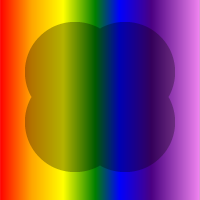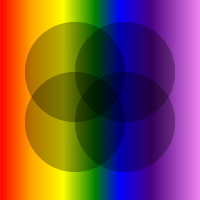Below is the corresponding AspPDF script:

Set PDF = Server.CreateObject("Persits.PDF")
Set Doc = PDF.CreateDocument

' Rainbow background image
Set Image = Doc.OpenImage( Server.MapPath( "17_rainbow.png" ) )

Set GState = Doc.CreateGState("blendmode=2")
Set GState2 = Doc.CreateGState("blendmode=1")

Call DrawCircles( 600, True, True )
Call DrawCircles( 400, True, False )
Call DrawCircles( 200, False, True )
Call DrawCircles( 0, False, False )

' Save document, the Save method returns generated file name
Filename = Doc.Save( Server.MapPath("groups.pdf"), False )

' Y-shift, isolated flag, knockout flag
Sub DrawCircles( Y, Isolated, Knockout )
Set Graph = Doc.CreateGraphics("left=0; bottom=0; right=200; top=200")

' Create a circle graphics
Set Circle = Doc.CreateGraphics("left=0; bottom=0; right=100; top=100")
Circle.Canvas.SetFillColor 0.7, 0.7, 0.7
Circle.Canvas.FillEllipse 50, 50, 50, 50

' Parameter object for group creation
Set Param = PDF.CreateParam
Param("Group") = 1 ' True
If Isolated Then Param("Isolated") = 1
If Knockout Then Param("Knockout") = 1
Set Group = Doc.CreateGState(Param)

Graph.SetGroup Group
Graph.Canvas.SetGState GState
Graph.Canvas.DrawGraphics Circle, "x=25; y=28"
Graph.Canvas.DrawGraphics Circle, "x=75; y=28"
Graph.Canvas.DrawGraphics Circle, "x=25; y=78"
Graph.Canvas.DrawGraphics Circle, "x=75; y=78"

Page.Canvas.SaveState
Page.Canvas.DrawImage Image, "x=0, y=" & Y
Page.Canvas.SetGState GState2
Page.Canvas.DrawGraphics Graph, "x=0; y=" & Y
Page.Canvas.RestoreState
End Sub
IPdfDocument objDoc;
IPdfPage objPage;
IPdfManager objPdf;
IPdfGState objGState;
IPdfGState objGState2;
IPdfImage objImage;

{
// Common part: draw background
objPdf = new PdfManager();
objDoc = objPdf.CreateDocument( Missing.Value );
objPage = objDoc.Pages.Add( Missing.Value, Missing.Value, Missing.Value );

// Rainbow background image
objImage = objDoc.OpenImage( Server.MapPath( "17_rainbow.png" ), Missing.Value );

objGState = objDoc.CreateGState("blendmode=2");
objGState2 = objDoc.CreateGState("blendmode=1");

DrawCircles( 600, true, true );
DrawCircles( 400, true, false );
DrawCircles( 200, false, true );
DrawCircles( 0, false, false );

// Save document, the Save method returns generated file name
string strFilename = objDoc.Save( Server.MapPath("groups.pdf"), false );
}

// Y-shift, isolated flag, knockout flag
void DrawCircles( int Y, bool Isolated, bool Knockout )
{
IPdfGraphics objGraph = objDoc.CreateGraphics("left=0; bottom=0; right=200; top=200");

// Create a circle graphics
IPdfGraphics objCircle = objDoc.CreateGraphics("left=0; bottom=0; right=100; top=100");
objCircle.Canvas.SetFillColor( 0.7f, 0.7f, 0.7f );
objCircle.Canvas.FillEllipse( 50, 50, 50, 50 );

// Parameter object for group creation
IPdfParam objParam = objPdf.CreateParam(Missing.Value);
objParam["Group"].Value = 1; // true
if( Isolated ) objParam["Isolated"].Value = 1;
if( Knockout ) objParam["Knockout"].Value = 1;
IPdfGState objGroup = objDoc.CreateGState(objParam);

objGraph.SetGroup( objGroup );
objGraph.Canvas.SetGState( objGState );
objGraph.Canvas.DrawGraphics( objCircle, "x=25; y=28" );
objGraph.Canvas.DrawGraphics( objCircle, "x=75; y=28" );
objGraph.Canvas.DrawGraphics( objCircle, "x=25; y=78" );
objGraph.Canvas.DrawGraphics( objCircle, "x=75; y=78" );

objPage.Canvas.SaveState();
objPage.Canvas.DrawImage( objImage, "x=0, y=" + Y );
objPage.Canvas.SetGState( objGState2 );
objPage.Canvas.DrawGraphics( objGraph, "x=0; y=" + Y );
objPage.Canvas.RestoreState();
}

Click the links below to run this code sample:

Any PDF compositing operation can be subject to a soft mask, or alpha mask, which is a graphics object that serves as the source of variable opacity for the objects being drawn. The word soft emphasizes that the mask value at a given point is not limited to just 0.0 or 1.0 but can take on intermediate fractional values as well. A soft mask is activated by linking it to a graphics state object, and then activating that object.

For a graphics object to act like a soft mask, it has to be associated with a transparency group. The soft mask values (opacity) can be derived either from the group's alpha values or from the group's luminosity values. In the latter case, the transparency group must be associated with a color space so that the luminocity could be properly calculated.

With AspPDF, a soft mask is created by creating an instance of the PdfGraphics object and associating it with a transparency group via the PdfGraphics.SetGroup method. The PdfGraphics object is then associated with a graphics state object via the PdfGState.SetSoftMask method. The SetSoftMask method expects two arguments: an instance of the PdfGraphics object and a parameter list. The optional Alpha parameter specifies whether the soft mask is to be derived from the group's alpha values (if set to True) or luminocity values (if set to False or omitted). The optional backdrop color for the group can also be specified via the C1, C2, ..., Cn color components (black by default.)

Note that if Alpha is False or omitted, the transparency group associated with this PdfGraphics object must be assigned a color space via the SetColorSpace method.

The following example uses a soft mask defined by a black and white image to create a drop shadow for a logo:Below is the corresponding AspPDF script:

Set PDF = Server.CreateObject("Persits.PDF")
Set Doc = PDF.CreateDocument

' Main Logo
Set Logo = Doc.CreateGraphics("left=0; bottom=0; right=128; top=206")
With Logo.Canvas
.SetParams ("FillColor=#313570")
.MoveTo 6, 177
.LineTo 48, 201
.LineTo 113, 162
.LineTo 113, 86
.LineTo 70, 62
.LineTo 70, 137
.ClosePath
.Fill

.SetParams ("FillColor=#5B88B1")
.MoveTo 61, 5
.LineTo 61, 130
.LineTo 20, 106
.LineTo 20, 30
.ClosePath
.Fill
End With

' Transparency group to be used with the soft mask
Set Group = doc.CreateGState("Group=true")
' Required as we use luminocity for soft mask
Group.SetColorSpace Doc.CreateColorSpace("DeviceRGB")

' Soft mask based on an image to be used as alpha
Set Image = Doc.OpenImage(Server.MapPath("17_alpha.png"))
Set SM = Doc.CreateGraphics("left=0; bottom=0; right=128; top=206")
SM.Canvas.DrawImage Image, "x=0; y=0"

' Associate the soft mask with a transparency group
SM.SetGroup Group

Set GState = Doc.CreateGState("fillalpha=0.5")
Set Shadow = Doc.CreateGraphics("left=0; bottom=0; right=128; top=206")
.SetGState GState
.SetFillColor 0.2, 0.2, 0.2 ' gray
.FillRect 0, 0, 128, 206
End With

' Draw shadow with an offset

' Draw logo
Page.Canvas.DrawGraphics Logo, "x=100; y=500"

' Save document, the Save method returns generated file name
Filename = Doc.Save( Server.MapPath("dropshadow.pdf"), False )
IPdfManager objPdf = new PdfManager();
IPdfDocument objDoc = objPdf.CreateDocument( Missing.Value );
IPdfPage objPage = objDoc.Pages.Add( Missing.Value, Missing.Value, Missing.Value );

// Main Logo
IPdfGraphics objLogo = objDoc.CreateGraphics("left=0; bottom=0; right=128; top=206");

objLogo.Canvas.SetParams("FillColor=#313570");
objLogo.Canvas.MoveTo(6, 177);
objLogo.Canvas.LineTo(48, 201);
objLogo.Canvas.LineTo(113, 162);
objLogo.Canvas.LineTo(113, 86);
objLogo.Canvas.LineTo(70, 62);
objLogo.Canvas.LineTo(70, 137);
objLogo.Canvas.ClosePath();
objLogo.Canvas.Fill(Missing.Value);

objLogo.Canvas.SetParams("FillColor=#5B88B1");
objLogo.Canvas.MoveTo(61, 5);
objLogo.Canvas.LineTo(61, 130);
objLogo.Canvas.LineTo(20, 106);
objLogo.Canvas.LineTo(20, 30);
objLogo.Canvas.ClosePath();
objLogo.Canvas.Fill(Missing.Value);

// Transparency group to be used with the soft mask
IPdfGState objGroup = objDoc.CreateGState("Group=true");
// Required as we use luminocity for soft mask
objGroup.SetColorSpace( objDoc.CreateColorSpace("DeviceRGB", Missing.Value ));

// Soft mask based on an image to be used as alpha
IPdfImage objImage = objDoc.OpenImage(Server.MapPath("17_alpha.png"), Missing.Value );
IPdfGraphics objSM = objDoc.CreateGraphics("left=0; bottom=0; right=128; top=206");
objSM.Canvas.DrawImage( objImage, "x=0; y=0" );

// Associate the soft mask with a transparency group
objSM.SetGroup( objGroup );

IPdfGState objGState = objDoc.CreateGState("fillalpha=0.5");
IPdfGraphics objShadow = objDoc.CreateGraphics("left=0; bottom=0; right=128; top=206");

objShadow.Canvas.SetFillColor( 0.2f, 0.2f, 0.2f ); // gray
objShadow.Canvas.FillRect( 0, 0, 128, 206 );

// Draw shadow with an offset

// Draw logo
objPage.Canvas.DrawGraphics( objLogo, "x=100; y=500" );

// Save document, the Save method returns generated file name
string strFilename = objDoc.Save( Server.MapPath("dropshadow.pdf"), false );

## 19.5 Miscellaneous Features

19.5.1 Page Groups

In all the transparency group-related examples of this chapter, a group was implemented by a PdfGraphics object. A group can also be represented by a PdfPage object as a whole, in the exact same manner. To have a page represent a group, the method PdfPage.SetGroup must be called, for example:

Set Group = Doc.CreateGState("Group=True; Isolated=True")